# Test: Estimation And Rounding- 2

## 20 Questions MCQ Test Quantitative Aptitude for GMAT | Test: Estimation And Rounding- 2

Description
Attempt Test: Estimation And Rounding- 2 | 20 questions in 40 minutes | Mock test for GMAT preparation | Free important questions MCQ to study Quantitative Aptitude for GMAT for GMAT Exam | Download free PDF with solutions
QUESTION: 1

### At a furniture maker, the cost of manufacturing a type of chair varies between \$100 and \$150, depending on the current prices of raw materials. The selling price of the chair varies between \$300 and \$400, depending on the availability of seasonal discounts. The profit percentage earned by the furniture maker on the sale of a chair of this type must be between:

Solution:

Given

• Let the Cost Price of the chair be CP and the Selling Price be SP
• 100 ≤ CP ≤ 150
• 300 ≤ SP ≤ 400

To Find:  The maximum and minimum percentage profit

Approach: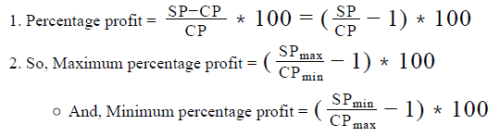Working out :

• Calculating maximum percentage profit
• Maximum SP = 400
• Minimum CP = 100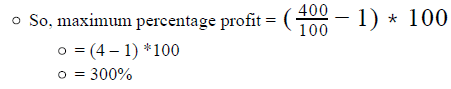• Calculating minimum percentage profit
• Minimum SP = 300
• Maximum CP = 150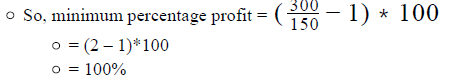Looking at the answer choices, we see that the correct answer is Option B

QUESTION: 2

### In a country X, length is measured in the units of sycras. If 1 sycra  is equal to 3.24 inches and there are 0.083 feet in 1 inch, which of the following best approximates the height of a 5 feet 3 inches tall woman in sycras?

Solution:

Given:

• 1 sycra = 3.24 inches
• 1 inch = 0.083 feet
• The height of a woman = say, H = 5 feet 3 inches

To Find: The approximate value of H in sycras

Approach:

1. Since the question asks only for an approximate value of H in sycras, we can use the principles of estimation and rounding to solve the question.
1. Since options B – D are fairly close in magnitude, we will take care to not estimate or round too aggressively.
2. Since we are given the relation between sycras and inches, if we can express H in inches, we will be able to express H in sycras.
3.  We are also given the relation between foots and inches. We’ll use that relation to convert the value of H (currently given in feet and inches) into inches.

Working out:

• Converting H from feet into inches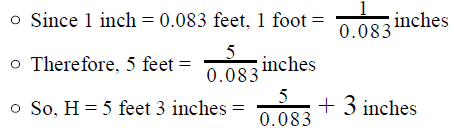• Converting H from inches into sycras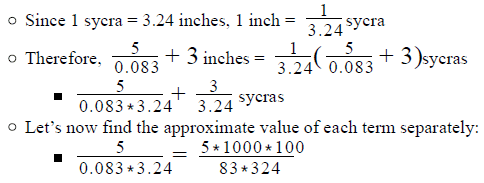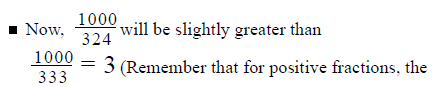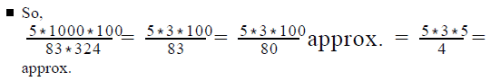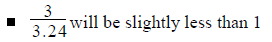• Therefore, the approximate value of the sum of the 2 terms will be slightly less than 18.75 + 1 = 19.75
So, we’ve seen that the height of the woman in sycras will be slightly less than 19.75
Looking at the answer choices, we see that the answer choice that is the closest to 19.75 is Option D
QUESTION: 3

### S is the sum of the reciprocals of the squares of the prime numbers between 19 and 41, exclusive. Which of the following is closest to the value of S?

Solution:

Given

Prime numbers between 19 and 41, exclusive = {23, 29, 31, 37}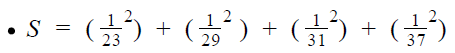To Find: The option that is closest to the value of S

Approach:

1. We’ll use the principles of estimation to answer the question.
1. Note that the 5 options are not very far – off in magnitude (for example, Options C and D are of the same order, 10 ). So, we’ll not approximate aggressively.
• To be on the safer side, we can estimate the Upper Bounds and the Lower Bounds for the value of S, so that we can then mark the answer we get with absolute confidence (and not worry if our answer could be wrong because we approximated more aggressively than we should have)

Working out

• Finding the Upper Bound for S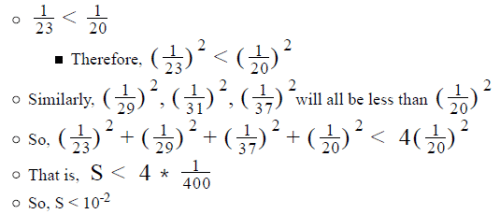• Note that just calculating the Upper Bound eliminates Options C – E

• Finding the Lower Bound for S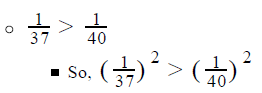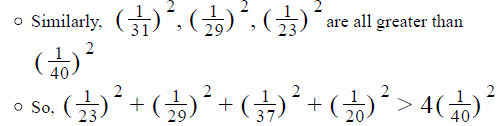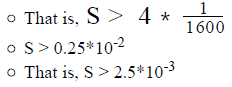• We’ve calculated above that 2.5*10-3 < S < 10*10-3

Looking at the answer choices, we see that the only option that satisfies this range is Option B

QUESTION: 4

Nick appeared in a test of quantitative ability that consisted of 100 questions. Nick was awarded 4 marks for each correct answer and 1 mark was deducted for each wrong answer. If the number of questions attempted by Nick was between 70 and 80, inclusive, and his accuracy range was 60% - 75%, inclusive, in what range did Nick’s score lie?

Solution:

Given:

• Number of questions = 100
• Correct answer = 4 marks
• Incorrect answer = -1 marks
• 70 ≤ Number of questions attempted ≤ 80
• 60% ≤ Accuracy ≤ 75%

To Find: Range of Nick’s score?

Approach

1. To find the range of Nick’s score, we need to find the maximum and minimum possible score of Nick
2. Maximum Possible Score
1. Nick’s score would be maximum when he attempts the maximum number of questions and with the highest accuracy rate.
3. Minimum Possible Score
1. Nick’s score would be minimum when he attempts the minimum number of questions and with the lowest accuracy rate

Working out:

1. Calculating the Maximum Possible Score

a. Maximum number of questions that Nick could attempt = 80
b. Highest accuracy rate = 75%
c. Number of questions that Nick got correct = 75% of 80 = 60
d. Number of questions that Nick got incorrect = 25% of 80 = 20
e. Total marks = 60 * 4 – 20 * 1 = 220

2. Calculating the Minimum Possible Score

a. Minimum number of questions that Nick could attempt = 70
b. Lowest accuracy rate = 60%

c. Number of questions that Nick got correct = 60% of 70 = 42
d. Number of questions that Nick got incorrect = 40% of 70 = 28
e. Total marks = 42 * 4 – 28 * 1 = 140

3. So, Nick’s scores would range between 140 – 220, inclusive

QUESTION: 5

If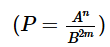, where A is a positive integer lesser than or equal to 20, B is a non-zero integer between -10 and 10, inclusive, and m and n are non-negative single-digit numbers, which of the following expressions is true?

Solution:

Given:

• Possible values of Integer A = {1, 2, 3 . . . 19, 20}
• Possible values of Integer B = { -10, -9, -8 . . . -1, 1, 2, 3 . . . 9, 10}
• B ≠ 0
• Possible values of m, n = {0, 1, 2, 3 . . . 8, 9}
•To Find: Out of the given options on the range of P, which is correct?

Approach:

• To answer this question, we need to find the maximum possible value of P and the minimum possible value of P
• P is maximum when An is maximum and B2m is minimum
• Since both A and n are non-negative integers, An will be maximum when A is maximum and n is maximum
• Since both B and m are non-negative integers, B2m will be minimum when both B and m are at their minimum possible values
• P is minimum when An is minimum and B2m is maximum
• Since both A and n are non-negative integers, A will be minimum when An is minimum and n is minimum
• Since both B and m are non-negative integers, B2m will be maximum when both B and m are at their maximum possible values.
• The option that lies between the calculated maximum and minimum values of P will be the correct answer

Working out:

• Calculating the maximum possible value of P
• Finding maximum value of An
• Maximum possible value of A = 20
• And, maximum possible value of n = 9
• So, maximum A = 20
• Finding minimum value of B2m
• Since this term is the perfect square of integer B, it’s least value can be 1 (happens when m = 0)
• Note that the minimum possible value of B did not depend on the value of B here only because the minimum possible value of m here is 0. Had m been a positive integer, then the minimum possible value of B would have depended on the minimum possible value of both m and B.
• So, P maximum =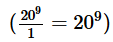• Calculating the minimum possible value of P
• Finding minimum value of An
• Minimum possible value of A = 1
• For this value of A, the value of n will not matter
• Note that the minimum possible value of A here didn’t depend on the value of n only because the minimum possible value of A was 1 (and 1 raised to the power of anything is equal to1). If however A had been a positive integer greater than 1, then to find the minimum possible value of A , you would have needed to consider the minimum values of both A and n.
• So, minimum A = 1
• Finding maximum value of B2m
• Maximum possible value of B = 10
• And, maximum possible value of m = 9
• So, maximum B2m = 1018
• So, P minimum =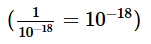• We’ve calculated that: 10-18 < P < 209

Looking at the answer choices, we see that the correct answer is Option C

QUESTION: 6

The sum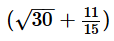is between

Solution:

Given:

The sumTo Find: The value of this sum lies between which of the 5 ranges given in the answer choices

Approach:

1. To find which of the 5 ranges the sum lies in, we need to know the approximate value of the sum.
2. To find the approximate value of the sum of the 2 terms, we will use the principles of estimation to first find the approximate value of √30 and of  11/5

Working out: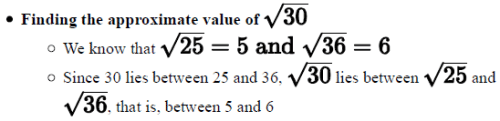• Further, note that 30 lies almost mid-way between 25 and 36.

Therefore,√30 will almost lie mid-way between 5 and 6

• So, we can say that the value of √30 = 5.5 approximately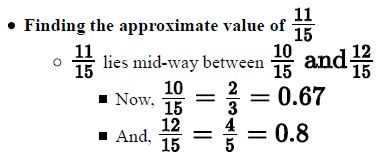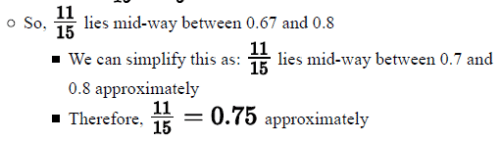• Finding the approximate value of the sum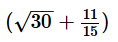• We’ve worked out above that the approximate value of: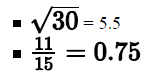• So, the approximate value of their sum = 5.5 + 0.75 = 6.25

Looking at the answer choices, we see that the correct answer is Option B

QUESTION: 7

If R and S are three-digit positive integers, what is the quotient when the sum of R and S is divided by 1000?
(1) The hundreds digit of the sum of R and S is less than the sum of the hundreds digits of R and S.
(2) When R, rounded to the nearest hundreds, is added to S, rounded to the nearest hundreds, the result is 1000.

Solution:

Step 1 & 2: Understand Question and Draw Inference

• Let R = 100a + 10b + c, where a, b and c are digits and a is non-zero (because if a is zero then R would be a 2-digit number)
• Let S = 100d + 10e + f, where d, e and f are digits and d is non-zero (because if d is zero then S would be a 2-digit number)

To find: The quotient when R + S is divided by 1000

• Now, R + S = 100(a+d) + 10(b+e) + (c+f)
• There are 2 possibilities – either the sum R + S will also be a 3-digit number (the case of n0 carry-over at the hundreds digit) or the sum R + S will be a 4-digit number (the case of carry–over at the hundreds digit). Let’s consider both these cases one-by-one:
• Case 1: The case of no carry-over
• The sum R+S is a 3-digit number which is divided by 1000 (for example, think of 900 or 990 being divided by 1000)
• In this case, the quotient when R+S is divided by 1000 is zero.
• Case 2: The case of carry-over
• The sum R+S is a 4-digit number here which is divided by 1000 (for example, think of 1800 or 1980 being divided by 1000)
• The quotient in this case will be 1
• If you’re wondering if the quotient can be greater than 1 in this case, the answer is, No it cannot be. The maximum value of any digit is 9. So, the maximum sum of 2 digits can be 18 (8 stays at the place of the digit and 1 gets carried over). So, the maximum carry-over that can happen when 2 digits are added, is 1.
• So, to answer the question, we need to determine if there is a carry-over at the hundreds digit or not.

Step 3 : Analyze Statement 1 independent

The hundreds digit of the sum of R and S is less than the sum of the hundreds digits of R and S.
There are 4 possible cases for the hundreds digit of R+S:
Case A: There is no carry-over into the hundreds digit and there is no carry-over at the hundreds digit
Example: 1 2 3
+ 2 3 4
In this example, R + S = 357
So, (hundreds digit of R+S) = 3
And (hundreds digit of R) + (hundreds digit of S) = 1 + 2 = 3
In this case, (hundreds digit of R+S) = (hundreds digit of R) + (hundreds digit of S)

Case B: There is no carry-over into the hundreds digit but there is carry-over happening AT the hundreds digit
Example: 9 2 3
+ 1 3 4
In this example, R + S = 1057
So, (hundreds digit of R+S) = 0
But (hundreds digit of R) + (hundreds digit of S) = 9 + 1 = 10
So, here, (hundreds digit of R+S) < (hundreds digit of R) + (hundreds digit of S)

Case C: There is carry-over into the hundreds digit and there is no carry-over AT the hundreds digit
Example: 7 9 4
+ 1 2 2
In this example, R + S = 916
So, (hundreds digit of R + S) = 9
Whereas, (hundreds digit of R) + (hundreds digit of S) = 7 + 1 = 8
In this case, (hundreds digit of R+S) > (hundreds digit of R) + (hundreds digit of S)

Case D: There is carry-over into the hundreds digit and there is carry-over happening AT the hundreds digit
Example 1: 6 9 4
+ 3 2 1
In this example, R + S = 1015
So, (hundreds digit of R + S) = 0
Whereas, (hundreds digit of R) + (hundreds digit of S) = 6 + 3 = 9
Here, (hundreds digit of R+S) < (hundreds digit of R) + (hundreds digit of S)

Example 2: 8 9 4
+ 7 2 1
In this example, R + S = 1615
So, (hundreds digit of R + S) = 6
Whereas, (hundreds digit of R) + (hundreds digit of S) = 15
Here too, (hundreds digit of R+S) < (hundreds digit of R) + (hundreds digit of S)

Therefore, we notice that in all the cases where Statement 1 is satisfied, that is the cases where (hundreds digit of R+S) < (hundreds digit of R) + (hundreds digit of S), there is carry-over is happening at the hundreds digit. Therefore, we can be sure that Case 2 discussed in ‘Steps 1 and 2’ is applicable. So, the quotient when R + S is divided by 1000 is 1.
Since we get a unique answer from this Statement, Statement 1 is sufficient to answer the question.

Step 4 : Analyze Statement 2 independent

When the R, rounded to the nearest hundreds, is added to S, rounded to the nearest hundreds, the result is 1000.

• R = 100a + 10b + c
• The result of rounding R to the nearest hundreds is:
• Either 100a
• Happens when the digit to the right of a, that is b, is less than 5
• Or 100(a+1)
• Happens when b ≥ 5
• S = 100d + 10e + f
• The result of rounding S to the nearest hundreds is:
• Either 100d
• Happens when e < 5
• Or 100(d+1)
• Happens when e ≥ 5
• As per Statement 2, (Result of rounding R to hundreds) + (Result of rounding S to hundreds) = 1000
• So, the following cases are possible:
• Case 1: 100a + 100d = 1000
• That is, a + d = 10
• So, in this case, carry-over will happen (in the sum R + S) into the thousands digit.
• Therefore, the quotient in this case will be 1

• Case 2: 100a + 100(d+1) = 1000
• That is, a + d + 1 = 10
• So, a + d = 9
• In this case, carry-over into the thousands digit may or may not happen in the sum R + S (depending on the value of b + e)
• For example, in the sum of 712 + 213, carry-over doesn’t happen into the thousands digit.
• However, in the sum of 782 + 293, carry-over does happen into the thousands digit. This is because the sum of the tens digit (8 + 9) results in a carry-over into the hundreds digit first, therefore, making the sum of the hundreds digit equal to 7 + 2 + 1, that is, 10. The 0 stays at the hundreds place and the 1 gets carried over into the thousands digit.
• So, the quotient may be 0 or 1.

• Case 3: 100(a+1) + 100d = 1000
• That is, a + d = 9
• This is the same equation as the one obtained in Case 2 above.
• So, here too, we may get either 0 or 1 as the quotient.

• Case 4: 100(a+1) + 100(d+1) = 1000
• That is, a + d + 2 = 10
• So, a + d = 8
• As we’ve discussed above, the maximum carry-over that may happen into the hundreds (or any digit for that matter) digit is 1. Even if a carried-over 1 is added to the sum of a + d, the new sum of a + d will still be 9 only. So, there will be no carry-over into the thousands digit.
• So, the quotient in this case will be 0

Thus, we’ve seen that from Statement 2, the value of the quotient may be 0 or 1.
So, Statement 2 is not sufficient to get a unique value of the quotient.

Step 5: Analyze Both Statements Together (if needed)

Since we’ve got a unique answer in Step 3, this step is not required

QUESTION: 8

T = 2.z hours
If z denotes the tenths digit in the decimal representation of the time T that a car takes to cover 258 miles, what is the value of z?
(1) The speed of the car is 1 mile per minute, rounded to the nearest integer
(2) When T is rounded to the nearest 100 minutes, the result is 200.

Solution:

Step 1 & 2: Understand Question and Draw Inference

Given:

• z is a digit. So, possible values of z: {0, 1, 2, 3 . . . ,8, 9}
• Distance = 258 miles
• T = 2.z0 hours
•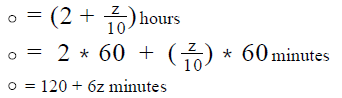• To find: z = ?

Step 3 : Analyze Statement 1 independent

• The speed of the car is 1 mile per minute, rounded to the nearest integer This means, 0.5 ≤ Actual speed (in miles per minute) < 1.5
• As per the formula for speed, Actual speed (in miles per minute) =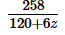•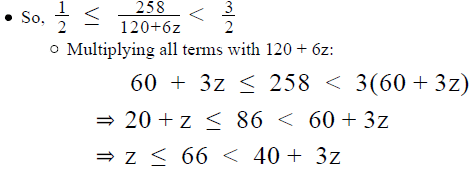• Thus, by simplifying the information given in Statement 1, we have arrived at 2 inequalities:Z ≤ 66 and  66 < 40 +3z. Let us now solve these 2 inequalities further, one by one
• Considering the 1 inequality: :
• Now, z is a digit. So, all possible values of z are less than 66. The first inequality therefore doesn’t help us find the value of z (because it doesn’t give us any new, useful information).
•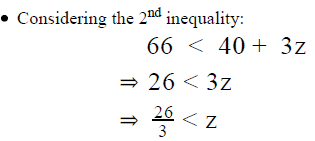• The above inequality can be rewritten as, Z > 26/3
• That is, z>8.67
• The only -possible value of z (remember that z is a digit) that is greater than 8.67 is 9.
• Thus, z = 9
Therefore, we’ve been able to determine a unique value of z from St. 1.

Step 4 : Analyze Statement 2 independent

• When T is rounded to the nearest 100 minutes, the result is 200.
• This means,150 ≤ T<250
• Substituting the given value of T in the above expression:
150 ≤ 120 + 6z < 250
30 ≤ 6z < 13
15 ≤ 3z < 65
• Considering the first part of the inequality: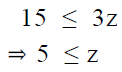• Thus, the possible values of z that satisfy the first part: {5, 6, 7, 8, 9}Considering the first part of the inequality: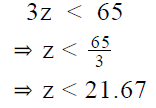• Since z is a digit, all possible values of z satisfy the 2 part of the inequality

Thus, from Statement 2, we get 5 possible values of z: {5, 6, 7, 8, 9}

So, Statement 2 is not sufficient to arrive at a unique answer.

Step 5: Analyze Both Statements Together (if needed)

We’ve already arrived at a unique answer in Step 3, so this step is not required Answer: Option A

QUESTION: 9

For every x celestial objects of the Sunny Way galaxy that can be seen from the Earth, there are x – (x) celestial objects of the galaxy that cannot be seen from the Earth. In the years of research done on the Sunny Way galaxy, scientists have been able to see only 93 planets in the galaxy. These planets were seen to have an average of 7 satellites per planet. It is also known that the ratio of the number of stars in the galaxy that are seen from the Earth to the number of satellites in the galaxy that are seen from the Earth is 2600:1 Assuming the same ratios are followed for the invisible celestial objects, which of the following is closest to the number of celestial objects in the Sunny Way galaxy?

Solution:

Given:

• For every x seen celestial objects, there are x3 – x unseen celestial objects
• Number of total objects = x + x3 – x = x3
• So, if the number of seen celestial objects is x, the number of total objects is x3
• 93 planets have been seen
• Average number of satellites per planet = 7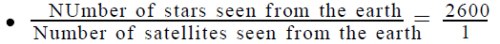To Find: Number of objects in the galaxy?

Approach:

1. Number of objects in the galaxy = number of stars + number of planets + number of satellites
2. Number of Planets
• As we know the number of planets seen from the earth, we can calculate the number of planets in the galaxy
3. Number of Satellites
• We are given the number of satellites per planet in the galaxy. As we know the number of planets seen, we can calculate the number of satellites seen.
• Once we calculate the number of satellites seen from the earth, we can calculate the number of satellites in the galaxy
4. Number of Stars
• ​​We are given the ratio of number of stars seen from the earth and the number of satellites seen from the earth. We can use this information to calculate the number of stars seen from the earth
• Once we know the number of stars seen from the earth, we can calculate the total stars in the galaxy.

Working out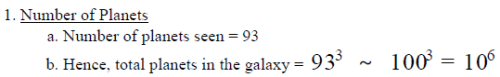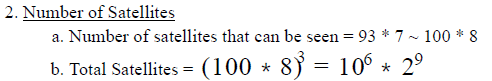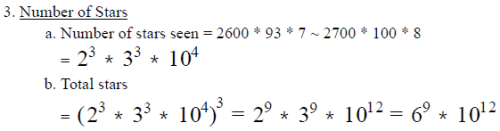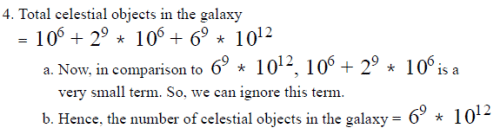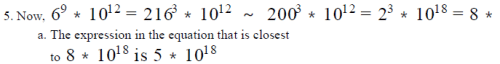QUESTION: 10

In a recurring manufacture – to- sale cycle, a toy manufacturer sells a type of toys to a retailer at a profit that varies between 50% and 60%, depending on the size of the retailer’s order (the retailer gets a proportional discount for bulk purchase of toys). The retailer marks up the price of these toys by 20% to 40% depending on the location of his different shops. The retailer then offers a discount, across all shops, of either 10% or \$15 on the purchase of a toy to the customer. If the manufacturer incurs a cost of \$100 to manufacture a toy, the profit earned by the retailer per toy must be between:

Solution:

Given:

• 50% ≤ Profit% of manufacturer ≤ 60%
• Cost Price of manufacturer = \$100
• 20% ≤ Mark-up percentage of the retailer ≤ 40%
• Discount = {10% or \$15 per toy}

To Find: Range of profit earned by the retailer

Approach:

1. To find the range of the profit of the retailer, we need to find the maximum and minimum profit earned by the retailer.
2. Maximum Profit earned = Maximum Selling Price – Corresponding Cost Price
3. Minimum Profit earned = Minimum Selling Price – Corresponding Cost Price
• As the mark-up price of the manufacturer is dependent on the cost price of the manufacturer and the selling price of the manufacturer is dependent on the mark-up price, for calculating the profit earned the selling price and the cost price will be dependent.
4. Cost Price of Retailer
• (Cost Price of retailer) = (selling price of manufacturer)
• Selling Price of Manufacturer depends upon the profit made by the manufacturer as the cost price of the manufacturer is fixed
• So, if the manufacturer makes the maximum profit, his selling price will be the maximum. And so, the retailer will have the maximum cost price
• If the manufacturer makes the minimum profit, his selling price will be the minimum. And so, the retailer’s cost price will be minimum
5. Selling Price of Retailer
Selling Price of the retailer will depend on the mark up% and the discount offered by the retailer.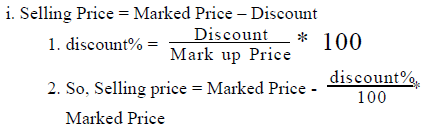ii.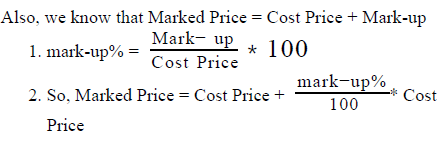iii. Selling price of the retailer will be maximum when the marked price is maximum and the discount offered is minimum

1. Marked price will be maximum when the mark-up% and the cost price is maximum
2. Marked price will be minimum when the mark-up% and the cost price is minimum

iv.  Selling price of the retailer will be minimum when the marked price is minimum and the discount offered is maximum

6. So, we see here that the maximum selling price of the manufacturer depends on the maximum mark-up price of the retailer, which is further dependent on the maximum cost price of the retailer.

1. Hence, maximum profit = Maximum selling price – maximum cost price
2. Similarly, minimum profit = Minimum selling price – minimum cost price

Working out

1. Cost Price of Retailer
• Cost Price of manufacturer = \$100
• Now, we know that Selling Price = Cost Price + Profit and profit% =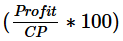• Combining the above two equations, we can write Selling price = Cost Price + Profit% * Cost Price
• Maximum Selling price of manufacturer = \$100 + maximum profit

i. Maximum profit% = 60%
ii. So, maximum selling price = 100 + 60% of 100 = 160
iii. So, maximum cost price of retailer = 160 …………..(1)

• Minimum Selling Price of manufacturer = \$100 + minimum profit

i. Minimum profit% = 50%
ii. So, minimum selling price = 100 +50% of 100 = 150
iii. So, minimum cost price of retailer = 150……………(2)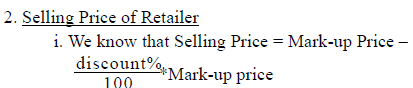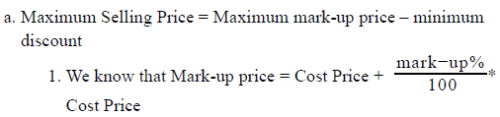1. Maximum marked price = Maximum cost price + maximum mark-up
1. Maximum marked price = 160 + 40% of 160 = \$224
2. So, maximum selling price = 224 – 15 = 209………(3)
3. So, we see that maximum selling price occurs for a cost price = \$160
4. Hence, maximum profit = 209 – 160 = \$49
• Minimum selling price = Minimum mark-up price – maximum discount
• Minimum marked price = minimum cost price + mark-up% * cost price
• Minimum marked price = 150 + 20% of 150 = \$180
• Minimum selling price = 180 – 10% of 180 = 162……..(4)
• So, we see that minimum selling price occurs for a cost price of \$150
• Hence, minimum profit = 162 – 150 = \$12

3. Hence, the profit ranges from \$12 -\$49

QUESTION: 11

A plot is in the shape of a right-angled triangle whose shorter sides measure 78.8 meters and 62.4 meters respectively. If an insect takes 1.79 minutes to cover a distance of 100 inches, what is the approximate time that it will take to return to its starting point after covering the entire perimeter of the plot in one direction? (1 meter is approximately 39.37 inches)

Solution:

Given:

• Insect’s speed = 100 inches in 1.79 minutes = 100 1.79 inches per minutes
• A right triangular plot:
• Shorter side 1, say x = 78.8 meters
• And, Shorter side 2, say y = 62.4 meters

To Find: Time taken by the insect to cover the perimeter of the plot

Approach:

1. Time = Distance / speed​
• So, to find the time, we’ll need to know the total distance (perimeter of the plot) that the insect covered
• Perimeter = x + y + Hypotenuse (let’s call it h henceforth)
• We already know the lengths of x and y. So, we’ll find h by using Pythagoras Theorem

• We are given the Speed of the insect.
• However, it’s important to note that since the side lengths of the plot are given in meters, our calculation of the Distance travelled by the insect will also be done in meters. However, the speed is given in units of inches per minute. So, when working out the solution, we must remember to:
• Either convert the Distance into inches
• Or convert the Speed into meters per minutes (or hours)

2. Also note the huge difference in the magnitude of different answer choices. This means, we can afford to use the principle of estimation and rounding aggressively to ease our calculations

Working out

• Finding the Distance travelled by the Insect
• We can round x to 80 and y to 60
• So,​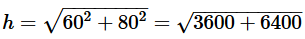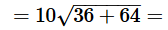• So, Distance travelled = Perimeter of triangle = 100 + 80 + 60 = 240 meters
• = 240*40 inches approx. (as 1 meter is equal to 39.37 inches)
• = 9600 inches approx.
• Finding the Time taken
• Speed = 100 1.79 inches per minutes
•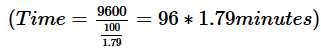• 100*1.79 minutes approx..
• = 179 minutes approx..
• = 3 hours approx..

Looking at the answer choices, we see that the correct answer is Option D

QUESTION: 12

The length and breadth of a rectangle are 2.4 units and 3.6 units respectively. If A is the area obtained by first multiplying the dimensions and then rounding the product to the nearest integer, and A* is the area obtained by first rounding each dimension to the nearest integer and then multiplying them, by what percentage is A* lesser or greater than A?

Solution:

Given:

• Length of rectangle = 2.4 units
• Breadth of rectangle = 3.6 units
• A = Result of rounding 2.4*3.6 to nearest integer
• A* = Product of (2.4 rounded to nearest integer) and (3.6 rounded to nearest integer)

To Find: By what percentage is A* less or greater than A?

Approach:

1. Let A* be p percentage greater than A. So, we can write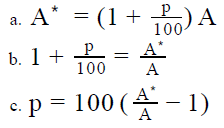d. So, to find the value of p, we need to find the value of A*/A
e. (Note: if upon calculation, the value of p comes to be negative, this will mean that A* is less than A by p percentage)

2. To find the value of A*/A, we’ll calculate the values of A* and A.

Working out :

• Finding the value of A*
• The result of rounding 2.4 to the nearest integer is 2
• The result of rounding 3.6 to the nearest integer is 4
• So, A* = 2*4 = 8
• Finding the value of A
• 2.4*3.6 = 8.64
• So, A = Result of rounding 8.64 to nearest integer
• That is, A = 9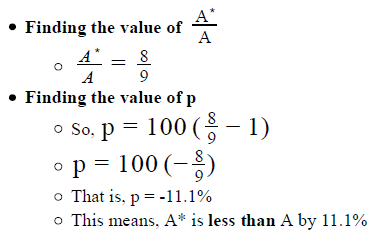Looking at the answer choices, we see that the correct answer is Option D

QUESTION: 13

If x is a positive integer, what is the tens digit of x?

1. When the product of 2 and (x+1) is rounded to the nearest tens, the result is 40
2. When the product of 3 and (x+1) is rounded to the nearest tens, the result is 70
Solution:

Step 1 & 2: Understand Question and Draw Inference

• x is an integer > 0

To Find: tens digit of x?

Step 3 : Analyze Statement 1 independent

1.  When the product of 2 and (x+1) is rounded to the nearest tens, the result is 40
• The integers that can rounded to the nearest tens and result in 40 ={35,36…….44}
• As 2(x+1) is even, 2(x+1) = { 36, 38, 40, 42, 44}
• So, x + 1 = {18, 19, 20, 21, 22}
• Therefore, x = { 17, 18, 19, 20, 21}
• Hence tens digit (x) = {1 or 2}

As we do not have a unique value of tens digit of x, the statement is insufficient to answer.

Step 4 : Analyze Statement 2 independent

2. When the product of 3 and (x+1) is rounded to the nearest tens, the result is 70

• The integers that can rounded to the nearest tens and result in 70 = {65, 66, …..73, 74}
• As 3(x+1) is a multiple of 3, the multiples of 3 in the above range are : { 66, 69, 72}
• So, x+1 = { 22, 23, 24}
• Hence tens digit(x) = 2

As we have a unique value of tens digit of x, this statement is sufficient to answer.

Step 5: Analyze Both Statements Together (if needed)

As we have a unique answer from step 4, this step is not required.

QUESTION: 14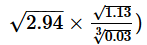is closest to which of the following

Solution:

Given:

•To Find: Approximate value of the expression

Approach:

1. As the expression is under square root and cube root, we will keep in mind the nearest squares or cubes of the terms under the root.
2. Also, we will try to use some basic algebraic functions to simplify the expression.

Working out: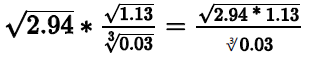1. Taking the expression in the numerator, which is under the root = 2.94 * 1.13 ~ 2.9 * 1.1 = (2+0.9) * (2-0.9) =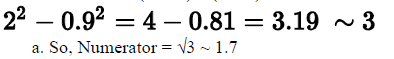2. Taking the expression in the denominator, which is under the root- 0.03 =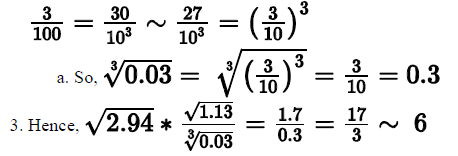QUESTION: 15

x = ab.cd, where a, b, c and d are the tens, units, tenths and hundredths digits respectively in the decimal representation of x. If the number obtained when x is rounded to the nearest integer is subtracted from the number 10a + b + 1, what is the result?

• 10a + b and 10c + d are prime numbers less than 50
• When the number is rounded to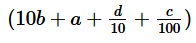the nearest integer, the result is 10b +a +1
Solution:

Step 1 & 2: Understand Question and Draw InferenceWhen x is rounded off to the nearest integer, the results can be:
I. 10a + b+ 1, if c ≥ 5
II. 10a + b , if c < 5

So, when the number obtained by rounding off x to the nearest integer is subtracted from 10a + b+ 1, we get:

• (10a + b + 1) – (10a + b + 1), that is 0, if c ≥ 5
• (10a + b + 1) – (10a + b), that is 1, if c < 5

Thus, we need to find if c ≥ 5 in order to find the required answer.

Step 3 : Analyze Statement 1 independent

1. 10a + b and 10c + d are prime numbers less than 50
As 10c + d < 50, c < 5.

Step 4 : Analyze Statement 2 independent

2. When the number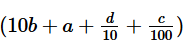is rounded to the nearest integer, the result is 10b +a +1
It tells us that d ≥ 5, however it does not tell us anything about c.

Step 5: Analyze Both Statements Together (if needed)

Since, we have a unique answer from step 4, this step is not required.

QUESTION: 16

In the third century BC, Greek mathematician and astronomer Eratosthenes calculated the circumference of the Earth to be 252,000 stadia. The stadion (plural: stadia) was a unit of length used in ancient Greece and the value of 1 stadion in terms of later units of length has been the subject of much debate. One belief is that 1 stadion is equivalent to 157.5 metres while another is that 1 stadion equals 185 metres. If the circumference of the Earth is now known to be 24,902 miles (where 1 mile is approximately 1.61 kilometres), depending upon the chosen equivalent measure of a stadion, the error in Erastothenes’ calculation is the closest to

Solution:

Given:

• Calculated circumference of Earth = 252,000 stadia
• 1 stadion = 157.5 meters or 185 meters
• Actual circumference of Earth = 24,902 miles
• 1 mile = 1.61 kilometer approx.

To Find: % error in Erastothenes’ calculation

Approach:

1.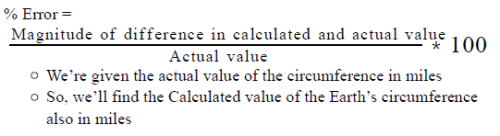2. We’ll do the calculation for each of the 2 possible conversions of 1 stadion into meters.

Working out:

• Calculating % Error for 1 stadion = 157.5 meters
• So, th​e Calculated circumference of Earth = 252,000*157.5 meters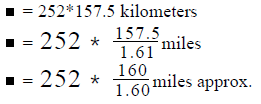• 252*100 miles approx.
• = 25200 miles approx

The Actual circumference of Earth = 24,902 miles = 24,900 miles approx.

(Looking at the answer choices, we’ll already know that the answer will be Option A, but still for the sake of completeness of the solution, we’ll also show below the calculation of % Error for the other possible value of 1 stadion)

• Calculating % Error for 1 stadion = 185 meters
• ​​So, the Calculated circumference of Earth = 252,000*185 meters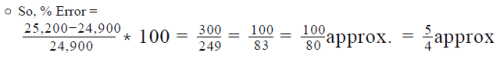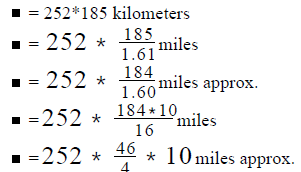• 63*460 miles approx
•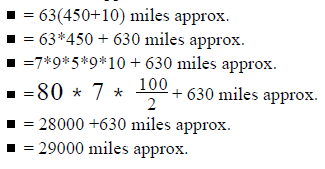• The Actual circumference of Earth = 24,902 miles = 24,900 miles approx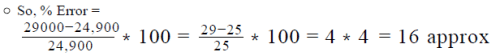• Evaluating the given answer choices
• Thus, depending on which conversion of stadion into meters is  chosen, the % error in Erastothenes’ calculation is either about 1% or about 16%

Looking at the answer choices, we see that the correct answer is Option A

QUESTION: 17

A company offers an allowance of \$500 perday to its employees for an official outstation visit. Pristine went on a two day official trip to Singapore. She made all trip-related expenses out of the allowance offered by the company and fully exhausted the allowance by the end of her trip. During the trip, she spent not less than \$350 and not more than \$400 each day on expenses other than food. If she had only 2 meals each day and paid a 15% tax and 5% tip on the price of each meal, the average price of her meal, excluding the tax and the tip, must be between:

Solution:

Given:

• Per day allowance offered = \$500
• Number of days in the trip = 2
• 350 ≤ Expense per day other than food ≤ 400
• Number of meals each day = 2
• Tax paid = 15%
• Tip = 5%
• To Find: Range of average price per meal excluding tax and tip?

Approach:

1. To find the average price per meal, we first need to find the maximum and minimum possible value of average amount spent on meal.
2. As we are given the total amount available to her and the amount spent on non-food items, we can find the maximum and minimum amount available to spend on food.
3. Once we find the maximum and minimum amount available to spend on food, we can reduce the tax and tip expenditure to find the cost of meal.
4. The, we will divide this cost with the total number of meals she took to find her average cost of meal

Working out:

1. Total amount available = \$500 * 2 = \$1000
2. Maximum amount spend on non-food items = \$400 * 2 = \$800
• So, minimum amount available to spend on food = \$1000 - \$800 = \$200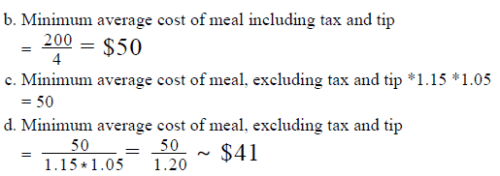3. Minimum amount spend on non-food items = \$350 *2 = \$700

a. So, maximum amount available to spend on food = \$1000 - \$700 = \$300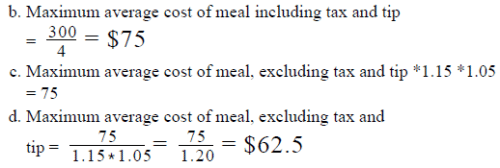d. Hence, the range of her average cost of meal was between \$41 and \$63

QUESTION: 18

z = 56.x43
In the decimal representation of z above, x denotes the tenths digit of z. Can x be equal to 2?
(1) When the product of 10 and z is rounded to the nearest integer, the result is 564
(2) When the product of 100 and z is rounded to the nearest integer, the result is 5644

Solution:

Step 1 & 2: Understand Question and Draw Inference

Given: Number z = 56.x43
To find: Can x be equal to 2? _______________

Step 3 : Analyze Statement 1 independent

Statement 1 says that ‘When the product of 10 and z is rounded to the nearest integer, the result is 564’

• 10z = 56x.43
• Result of rounding 56x.43 to the nearest integer = 56x (since the digit next to the place of rounding is 4 – which is less than 5 – the digit at the place of rounding will remain x)
• Upon comparing 56x with 564, we see that x = 4
• So, the answer to the question is NO

Statement 1 alone is sufficient to answer the question

Step 4 : Analyze Statement 2 independent

Statement 2 says that ‘When the product of 100 and z is rounded to the nearest integer, the result is 5644’

• 100z = 56x4.3
• Result of rounding 56x4.3 to the nearest integer = 56x4 (since the digit next to the place of rounding is 3 – which is less than 5 – the digit at the place of rounding will remain 4)
• Upon comparing 56x4 with 5644, we see that x = 4
• So, the answer to the question is NO

Statement 2 alone is sufficient to answer the question

Step 5: Analyze Both Statements Together (if needed)

Since we get a unique answer in each of Steps 3 and 4, this step is not required

QUESTION: 19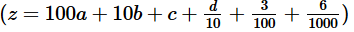If a, b, c and d denote the hundreds, tens, units and tenths digits respectively in the decimal representation of z above, is the number that results when z is rounded to the nearest integer divisible by 3?
(1) When  z100 is rounded to the nearest hundredths, the result is 1.85
(2) When  z +2 is rounded to the nearest tens, the result is 190

Solution:

Step 1 & 2: Understand Question and Draw Inference

Given:

•where a, b, c and d are digits

To find: When z is rounded to the nearest integer, is the result divisible by 3?

• The number that results when z is rounded to the nearest integer =
• 100a + 10b + c (When d < 5)
• Or 100a + 10b + (c + 1) (when d ≥ 5)
• So, the answer will be YES if:
• a + b + c is divisible by 3 (when d < 5)
• Or, a + b + c +1 is divisible by 3 (when d ≥ 5)

Step 3 : Analyze Statement 1 independent

•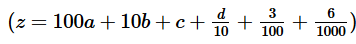• Result of rounding to nearest hundredths ( ) =
a.bc if d < 5
Or, a.b(c+1) if d ≥ 5
• Given in Statement 1: Result of rounding = 1.85
• If d < 5, then a.bc = 1.85
• a = 1, b = 8 and c = 5
• a + b + c = 1 + 8 + 5 = 14
• Thus, a + b + c is NOT divisible by 3
• So, the answer to the question is NO
• If d ≥ 5, then a.b(c+1) = 1.85
• a = 1, b = 8 and (c+1) = 5
• So, a + b + (c+1) = 1 + 8 + 5 = 14
• Thus, a + b + (c+1) is NOT divisible by 3
• So, the answer to the question is NO
• Thus, we’ve seen that in each of the 2 possible cases, we get the same answer to the asked question: NO

So, Statement 1 is sufficient to find a unique answer to the question.

Step 4 : Analyze Statement 2 independent

• When z + 2 is rounded to the nearest tens, the result is 190
• z+2 = ab(c+2).d36
• Result of rounding z+2 to nearest tens (ab (c+2).d36)=
• ab0 if c+2 < 5, that is, if c < 3
• Or, a(b + 1)0 if c+2 ≥ 5, that is, if c ≥ 3
• Given in Statement 2: Result of rounding = 190
• If c < 3, then ab0 = 190
• So, a = 1, b = 9
• Possible values of c = {0, 1, 2}
• So, possible values of the sum a + b + c = {10, 11, 12}
• Possible values of the sum a + b + (c+1) = { 11, 12, 13}
• (Note: Since we don’t know if d < 5 or not, we are considering the sum of digits in both cases: if d < 5 or if d ≥ 5)
• Thus, we see that in each of the 2 cases possible, the sum of the digits may or may not be divisible by 3
• Thus, the answer to the question may be YES or NO
• If c+2 ≥5, then a(b + 1)0 = 190
• So, a = 1, b = 8
• Possible values of c = {4, 5, 6, 7, 8 or 9}
• So, again, the sum (a + b + c) or (a + b + c + 1) may or may not be divisible by 3
• Thus, the answer to the question may be YES or NO

So, Statement 2 alone is not sufficient to answer the question

Step 5: Analyze Both Statements Together (if needed)

Since we’ve already arrived at a unique answer in Step 3, this step is not required

QUESTION: 20

n = 92.abc, where a, b and c denote the tenths, hundredths and thousandths digits respectively in the decimal representation of n.
When n is rounded to the nearest integer, the result is 92. If c is the average of two consecutive prime numbers such that c > b > a,
what is the difference between the maximum and minimum possible sum of a, b and c?

Solution:

Given:

• n = 92.abc
• a, b and c denote the tenths, hundredths and thousandths digits respectively
• Being digits of a number 0 ≤ a, b, c ≤ 9
• c > b > a
• Result of rounding n to the nearest integer = 92
•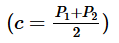, where P ,P are consecutive prime numbers
• To Find: Max(a + b + c) – Min(a + b + c)

Approach:

1. For finding the value of Max (a + b + c) – Min (a + b + c), we need to find the range of values for a, b and c
2. We know that the value of n when rounded to the nearest integer will depend on the value of a.
1. Since we are given that n, when rounded to the nearest integer is 92, we can find the range of values of n.
3. We know that c is the average of two consecutive prime numbers. As c ≤ 9, we can find the possible values of c.
4. Also, we will use the relation c > b > a, to find the possible values of b, because we already know the range of possible values of a and c.

Working out:

1. As n when rounded to the nearest integer = 92, a ≤ 4………….(1)

a. Maximum value of a = 4
b. Minimum value of a = 0

2. Since c is an integer ≤ 9 and c is the average of two consecutive prime numbers, possible values of c can be: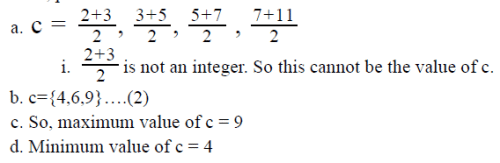3. Now, b is a digit from 0 to 9, however keeping in mind the constraint that b > a, b ≠ 0, because in that case b will not be greater than a.
a. So, Minimum value of b = 1 …….(3)
b. Also, since b < c, b ≠ 9, because in that case b will not be less than c.
c. So, maximum value of b = 8…….(4)

4. Combining (1), (2), (3) and (4), we have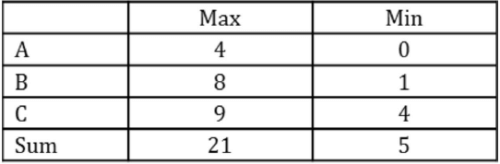5. Hence, Max (a + b + c) – Min (a+ b + c) = 21 – 5 = 16Use Code STAYHOME200 and get INR 200 additional OFF Use Coupon Code